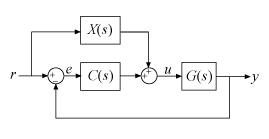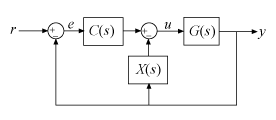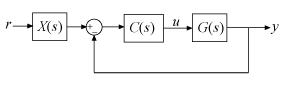Documentation

# getComponents

Extract SISO control components from a 2-DOF PID controller

## Syntax

``````[C,X] = getComponents(C2,looptype)``````

## Description

example

``````[C,X] = getComponents(C2,looptype)``` decomposes the 2-DOF PID controller `C2` into two SISO control components. One of the control components, `C`, is a 1-DOF PID controller. The other, `X`, is a SISO dynamic system. When `C` and `X` are connected in the loop structure specified by `looptype`, the resulting closed-loop system is equivalent to the 2-DOF control loop.For more information about 2-DOF PID control architectures, see Two-Degree-of-Freedom PID Controllers.```

## Examples

collapse all

Decompose a 2-DOF PID controller into SISO control components, using each of the feedforward, feedback, and filter configurations.

To start, obtain a 2-DOF PID controller. For this example, create a plant model and tune a 2-DOF PID controller for it.

```G = tf(1,[1 0.5 0.1]); C2 = pidtune(G,'pidf2',1.5)```
```C2 = 1 s u = Kp (b*r-y) + Ki --- (r-y) + Kd -------- (c*r-y) s Tf*s+1 with Kp = 1.12, Ki = 0.23, Kd = 1.3, Tf = 0.122, b = 0.664, c = 0.0136 Continuous-time 2-DOF PIDF controller in parallel form. ```

`C2` is a `pid2` controller object, with two inputs and one output. Decompose `C2` into SISO control components using the feedforward configuration.

`[Cff,Xff] = getComponents(C2,'feedforward')`
```Cff = 1 s Kp + Ki * --- + Kd * -------- s Tf*s+1 with Kp = 1.12, Ki = 0.23, Kd = 1.3, Tf = 0.122 Continuous-time PIDF controller in parallel form. Xff = -10.898 (s+0.2838) ------------------ (s+8.181) Continuous-time zero/pole/gain model. ```

As the display shows, this command returns the SISO PID controller `Cff` as a `pid` object. The feedforward compensator `X` is returned as a `zpk` object.

Decompose `C2` using the feedback configuration. In this case as well, `Cfb` is a `pid` controller object, and the feedback compensator `X` is a `zpk` model.

`[Cfb,Xfb] = getComponents(C2,'feedback');`

Decompose `C2` using the filter configuration. Again, the components are a SISO `pid` controller and a `zpk` model representing the prefilter.

`[Cfr,Xfr] = getComponents(C2,'filter');`

## Input Arguments

collapse all

2-DOF PID controller to decompose, specified as a `pid2` or `pidstd2` controller object.

Loop structure for decomposing the 2-DOF controller, specified as `'feedforward'`, `'feedback'`, or `'filter'`. These correspond to the following control decompositions and architectures:

• `'feedforward'``C` is a conventional SISO PID controller that takes the error signal as its input. `X` is a feedforward controller, as shown:If `C2` is a continuous-time, parallel-form controller, then the components are given by:

`$\begin{array}{c}C\left(s\right)={K}_{p}+\frac{{K}_{i}}{s}+\frac{{K}_{d}s}{{T}_{f}s+1},\\ X\left(s\right)=\left(b-1\right){K}_{p}+\frac{\left(c-1\right){K}_{d}s}{{T}_{f}s+1}.\end{array}$`

The following command constructs the closed-loop system from r to y for the feedforward configuration.

`T = G*(C+X)*feedback(1,G*C);`
• `'feedback'``C` is a conventional SISO PID controller that takes the error signal as its input. `X` is a feedback controller from y to u, as shown:If `C2` is a continuous-time, parallel-form controller, then the components are given by:

`$\begin{array}{c}C\left(s\right)=b{K}_{p}+\frac{{K}_{i}}{s}+\frac{c{K}_{d}s}{{T}_{f}s+1},\\ X\left(s\right)=\left(1-b\right){K}_{p}+\frac{\left(1-c\right){K}_{d}s}{{T}_{f}s+1}.\end{array}$`

The following command constructs the closed-loop system from r to y for the feedback configuration.

`T = G*C*feedback(1,G*(C+X));`
• `'filter'``X` is a prefilter on the reference signal. `C` is a conventional SISO PID controller that takes as its input the difference between the filtered reference and the output, as shown:If `C2` is a continuous-time, parallel-form controller, then the components are given by:

`$\begin{array}{c}C\left(s\right)={K}_{p}+\frac{{K}_{i}}{s}+\frac{{K}_{d}s}{{T}_{f}s+1},\\ X\left(s\right)=\frac{\left(b{K}_{p}{T}_{f}+c{K}_{d}\right){s}^{2}+\left(b{K}_{p}+{K}_{i}{T}_{f}\right)s+{K}_{i}}{\left({K}_{p}{T}_{f}+{K}_{d}\right){s}^{2}+\left({K}_{p}+{K}_{i}{T}_{f}\right)s+{K}_{i}}.\end{array}$`

The following command constructs the closed-loop system from r to y for the filter configuration.

`T = X*feedback(G*C,1);`

The formulas shown above pertain to continuous-time, parallel-form controllers. Standard-form controllers and controllers in discrete time can be decomposed into analogous configurations. The `getComponents` command works on all 2-DOF PID controller objects.

## Output Arguments

collapse all

SISO PID controller, returned as a `pid` or `pidstd` controller object. The form of `C` corresponds to the form of the input controller `C2`. For example, if `C2` is a standard-form `pidstd2` controller, then `C` is a `pidstd` object.

The precise functional form of `C` depends on the loop structure you specify with the `looptype` argument, as described in Input Arguments.

SISO control component, specified as a zero-pole-gain (`zpk`) model. The precise functional form of `X` depends on the loop structure you specify with the `looptype` argument, as described in Input Arguments.